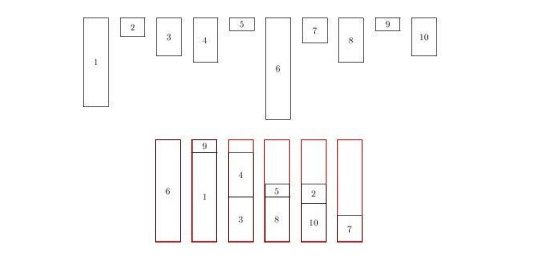# 悲催的移寝

Time limit:1000 ms Memory limit:131072 kB

1

10
80
70
15
30
35
10
80
20
35
10
30

6

### Hint### Code：

#include <iostream>
#include <algorithm>
#include <cstdio>
#include <cstring>
#define mem(a,b) memset(a,b,sizeof(a))
using namespace std;

const int maxn=1e5+5;
int a[maxn];

int main()
{
int T;
scanf("%d",&T);
while(T--)
{
int n,l;
scanf("%d%d",&n,&l);
for(int i=0;i<n;i++)
{
scanf("%d",a+i);
}
sort(a,a+n);

int cnt=0,len=n-1;
for(int i=0;a[i]&&i<n;i++)
{
while(a[len]+a[i]>l&&a[len]!=0)
{
len--;
}
if(a[len]+a[i]<=l&&a[len]!=0&&i!=len)
{
a[len]=0;
len--;
a[i]=0;
cnt++;
}
}
printf("%d\n",n-cnt);
if(T!=0)
printf("\n");
}

return 0;
}